Next: Atwood Machines Up: Lagrangian Dynamics Previous: Lagrange's Equation

Motion in a Central Potential

Consider a particle of mass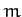moving in two dimensions in the central potential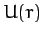. This is clearly a two degree of freedom dynamical system. As described in Section 5.5, the particle's instantaneous position is most conveniently specified in terms of the plane polar coordinates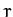and. These are our two generalized coordinates. According to Equation (223), the square of the particle's velocity can be written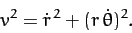(614)

Hence, the Lagrangian of the system takes the form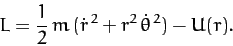(615)

Note that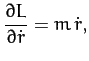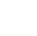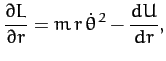(616)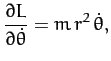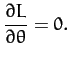(617)

Now, Lagrange's equation (613) yields the equations of motion,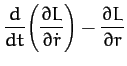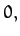(618)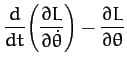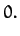(619)

Hence, we obtain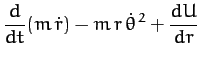(620)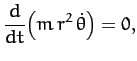(621)

or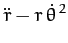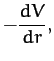(622)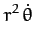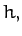(623)

where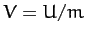, and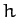is a constant. We recognize Equations (622) and (623) as the equations we derived in Chapter 5 for motion in a central potential. The advantage of the Lagrangian method of deriving these equations is that we avoid having to express the acceleration in terms of the generalized coordinatesand.Next: Atwood Machines Up: Lagrangian Dynamics Previous: Lagrange's Equation
Richard Fitzpatrick 2011-03-31